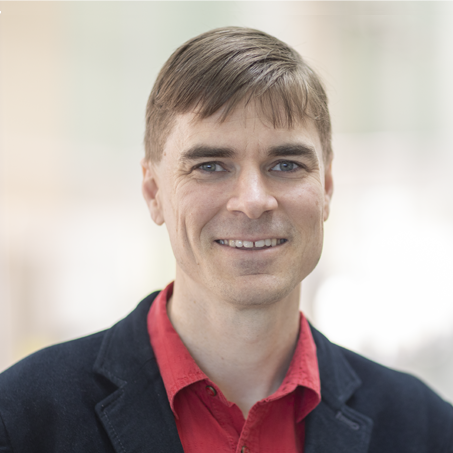Project Description

Riemann solvers are the core computation in some numerical methods for hyperbolic conservation laws. For such methods, the Riemann solvers are one of the most computationally demanding part of the entire algorithm. For this reason a common strategy is to develop efficient methods that approximate the solution of a Riemann problem.In recent years some highly efficient iterative algorithms have been proposed to approximate the solution of Riemann problems to arbitrary precision.This suggests that one could obtain the exact solution of Riemann problems (up to machine precision) in few iterations.As a result, one could attempt to consider the exact solution of Riemann problems instead of the more standard approximations without an excessive over head on computational cost.In this project we are interested on using state of the art exact Riemann solvers within the context of high-order finite volume methods and compare the overall quality, computational cost and robustness of the solution versus more standard approximate Riemann solvers. To do this we plan to concentrate on the Euler equations and the shallowwater model. ​​​Program - Applied Mathematics and Computer ScienceDivision - Computer, Electrical and Mathematical Sciences and EngineeringField of Study - ​Applied mathematics and computational science​

David I. Ketcheson

Associate Professor, Applied Mathematics and Computational Science​Professor Ketcheson's research interests are in the areas of numerical
analysis and hyperbolic PDEs. His work includes development of efficient
time integration methods, wave propagation algorithms, and modeling of
wave phenomena in heterogeneous media.

Desired Project Deliverables

​- Implementation of exact Riemann solvers for Euler and Shallow water equations, with high-accuracy initial guesses- Comparison of performance between exact and approximate Riemann solvers on a range of test problems, using PyClaw​